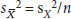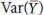Among the most common types of experiments are comparative studies that contrast outcomes under different conditions such as male versus female, placebo versus drug, or before versus after treatment. The analysis of these experiments calls for methods to quantitatively compare samples to judge whether differences in data support the existence of an effect in the populations they represent. This analysis is straightforward and robust when independent samples are compared; but researchers must often compare related samples, and this requires a different approach. We discuss both situations.

We'll begin with the simple scenario of comparing two conditions. This case is important to understand because it serves as a foundation for more complex designs with multiple simultaneous comparisons. For example, we may wish to contrast several treatments, track the evolution of an effect over time or consider combinations of treatments and subjects (such as different drugs on different genotypes).

We will want to assess the size of observed differences relative to the uncertainty in the samples. By uncertainty, we mean the spread as measured by the s.d., written as σ and s when referring to the population and sample estimate, respectively. It is more convenient to model uncertainty using variance, which is the square of the s.d. and denoted by Var() (or σ2) and s2 for the population and sample, respectively. Using this notation, the relationship between the uncertainty in the population of sample means and that of the population isfor samples of size n. The equivalent statement for sample data is, whereis the s.e.m. and sX is the sample s.d.

Recall our example of the one-sample t-test in which the expression of a protein was compared to a reference value1. Our goal will be to extend this approach, in which only one quantity had uncertainty, to accommodate a comparison of two samples, in which both quantities now have uncertainty. Figure 1a encapsulates the relevant distributions for the one-sample scenario. We assumed that our sample X was drawn from a population, and we used the sample meanto estimate the population mean. We defined the t-statistic (t) as the difference between the sample mean and the reference value, μ, in units of uncertainty in the mean, given by the s.e.m., and showed that t follows the Student's t-distribution1 when the reference value is the mean of the population. We computed the probability that the difference between the sample and reference was due to the uncertainty in the sample mean. When this probability was less than a fixed type I error level, α, we concluded that the population mean differed from μ.Figure 1: The uncertainty in a sum or difference of random variables is the sum of the variables' individual uncertainties, as measured by the variance.

Let's now replace the reference with a sample Y of size m (Fig. 1b). Because the sample means are an estimate of the population means, the differenceserves as our estimate of the difference in the mean of the populations. Of course, populations can vary not only in their means, but for now we'll focus on this parameter. Just as in the one-sample case, we want to evaluate the difference in units of its uncertainty. The additional uncertainty introduced by replacing the reference with Y will need to be taken into account. To estimate the uncertainty in, we can turn to a useful result in probability theory.

For any two uncorrelated random quantities, X and Y, we have the following relationship: Var(X − Y) = Var(X) + Var(Y). In other words, the expected uncertainty in a difference of values is the sum of individual uncertainties. If we have reason to believe that the variances of the two populations are about the same, it is customary to use the average of sample variances as an estimate of both population variances. This is called the pooled variance, sp2. If the sample sizes are equal, it is computed by a simple average, sp2 = (sX2 + sY2)/2. If not, it is an average weighted by n − 1 and m − 1, respectively. Using the pooled variance and applying the addition of variances rule to the variance of sample means gives. The uncertainty inis given by its s.d., which is the square root of this quantity.

To illustrate with a concrete example, we have reproduced the protein expression one-sample t-test example1 in Figure 2a and contrast it to its two-sample equivalent in Figure 2b. We have adjusted sample values slightly to better illustrate the difference between these two tests. For the one-sample case, we find t = 2.93 and a corresponding P value of 0.04. At a type I error cutoff of α = 0.05, we can conclude that the protein expression is significantly elevated relative to the reference. For the two-sample case, t = 2.06 and P = 0.073. Now, when the reference is replaced with a sample, the additional uncertainty in our difference estimate has resulted in a smaller t value that is no longer significant at the same α level. In the lookup between t and P for a two-sample test, we use d.f. = n + m − 2 degrees of freedom, which is the sum of d.f. values for each sample.Figure 2: In the two-sample test, both samples contribute to the uncertainty in the difference of means.

Our inability to reject the null hypothesis in the case of two samples is a direct result of the fact that the uncertainty inis larger than in(Fig. 1b) because nowis a contributing factor. To reach significance, we would need to collect additional measurements. Assuming the sample means and s.d. do not change, one additional measurement would be sufficient—it would decreaseand increase the d.f. The latter has the effect of reducing the width of the t-distribution and lowering the P value for a given t.

This reduction in sensitivity is accompanied by a reduction in power2. The two-sample test has a lower power than the one-sample equivalent, for the same variance and number of observations per group. Our one-sample example with a sample size of 5 has a power of 52% for an expression change of 1.0. The corresponding power for the two-sample test with five observations per sample is 38%. If the sample variance remained constant, to reach the 52% power, the two-sample test would require larger samples (n = m = 7).

When assumptions are met, the two-sample t-test is the optimal procedure for comparing means. The robustness of the test is of interest because these assumptions may be violated in empirical data. One way departure from optimal performance is reported is by the difference between α—the type I error rate we think we are testing at—and the actual type I error rate, τ. If all assumptions are satisfied, α = τ, and our chance of committing a type I error is indeed equal to α. However, failing to satisfy assumptions can result in τ > α, causing us to commit a type I error more often than we think. In other words, our rate of false positives will be larger than planned for. Let's examine the assumptions of the t-test in the context of robustness.

First, the t-test assumes that samples are drawn from populations that are normal in shape. This assumption is the least burdensome. Systematic simulations of a wide range of practical distributions find that the type I error rate is stable within 0.03 < τ < 0.06 for α = 0.05 for n ≥ 5 (ref. 3).

Next, sample populations are required to have the same variance (Fig. 1b). Fortunately, the test is also extremely robust with respect to this requirement—more so than most people realize3. For example, when the sample sizes are equal, testing at α = 0.05 (or α = 0.01) gives τ < 0.06 (τ < 0.015) for n ≥ 15, regardless of the difference in population variances. If these sample sizes are impractical, then we can fall back on the result that τ < 0.064 when testing at α = 0.01 regardless of n or difference in variance. When sample sizes are unequal, the impact of a variance difference is much larger, and τ can depart from a substantially. In these cases, the Welch's variant of the t-test is recommended, which uses actual sample variances, sX2/n + sY2/m, in place of the pooled estimate. The test statistic is computed as usual, but the d.f. for the reference distribution depends on the estimated variances.

The final, and arguably most important, requirement is that the samples be uncorrelated. This requirement is often phrased in terms of independence, though the two terms have different technical definitions. What is important is that their Pearson correlation coefficient (ρ) be 0, or close to it. Correlation between samples can arise when data are obtained from matched samples or repeated measurements. If samples are positively correlated (larger values in first sample are associated with larger values in second sample), then the test performs more conservatively (τ < α)4, whereas negative correlations increase the real type I error (τ > α). Even a small amount of correlation can make the test difficult to interpret—testing at α = 0.05 gives τ < 0.03 for ρ > 0.1 and τ > 0.08 for ρ < −0.1.

If values can be paired across samples, such as measurements of the expression of the same set of proteins before and after experimental intervention, we can frame the analysis as a one-sample problem to increase the sensitivity of the test.

Consider the two samples in Figure 3a, which use the same values as in Figure 2b. If samples X and Y each measure different sets of proteins, then we have already seen that we cannot confidently conclude that the samples are different. This is because the spread within each sample is large relative to the differences in sample means. However, if Y measures the expression of the same proteins as X, but after some intervention, the situation is different (Fig. 3b), now we are concerned not with the spread of expression values within a sample but with the change of expression of a protein from one sample to another. By constructing a sample of differences in expression (D; Fig. 3c), we reduce the test to a one-sample t-test in which the sole source of uncertainty is the spread in differences. The spread within X and Y has been factored out of the analysis, making the test of expression difference more sensitive. For our example, we can conclude that expression has changed between X and Y at P = 0.02 (t = 3.77) by testingagainst the null hypothesis that μ = 0. This method is sometimes called the paired t-test.